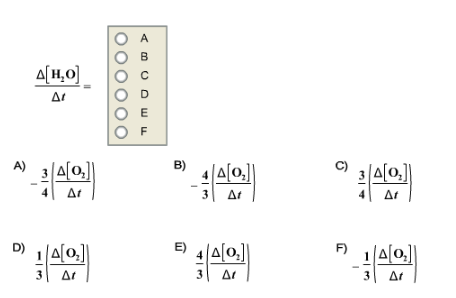# Problem: Given the following balanced chemical equation 3 O2 + 2 CH3OH → 2 CO2 + 4 H2OHow is the rate of appearance of H2O related to the rate of disappearance of O2?

###### FREE Expert Solution

Recall that for a reaction aA  bB, the rate of a reaction is given by:

$\overline{){\mathbf{Rate}}{\mathbf{=}}{\mathbf{-}}\frac{\mathbf{1}}{\mathbf{a}}\frac{\mathbf{\Delta }\mathbf{\left[}\mathbf{A}\mathbf{\right]}}{\mathbf{\Delta t}}{\mathbf{=}}\frac{\mathbf{1}}{\mathbf{b}}\frac{\mathbf{\Delta }\mathbf{\left[}\mathbf{B}\mathbf{\right]}}{\mathbf{\Delta t}}}$

where:

Δ[A] = change in concentration of reactants or products (in mol/L or M), [A]final – [A]initial

Δt = change in time, tfinal – tinitial

Reaction: 3 O2 + 2 CH3OH → 2 CO2 + 4 H2O

H2O is a product, the rate with respect to H2O is positive (+) since we’re gaining products.

O2 is a reactant, the rate with respect to O2 is negative (–) since we’re losing reactants.###### Problem Details

Given the following balanced chemical equation

3 O2 + 2 CH3OH → 2 CO2 + 4 H2O

How is the rate of appearance of H2O related to the rate of disappearance of O2